# Divisor and Dividend: Explained in Simple Words!Divisor and dividend are often confused by the students. Whether they’re learning math in their traditional face-to-face classes or taking extra sessions from an online tutor, students can’t skip this topic and jump to the easier one!

Divisor and dividend are the roots of the divisibility rule. Both terms sound familiar and even in math you always see them together. However, both expressions hold different meanings and are used to represent different terms.

In this blog, I’ve explained what divisors and dividends are in very simple words. You’ll also find examples down below.

Click on the “Table of Contents” button for getting a glimpse of the topics I’ve covered in this blog.

## What is Divisor?

The divisor is the number that is used to divide another number in the process of division. Here is an example: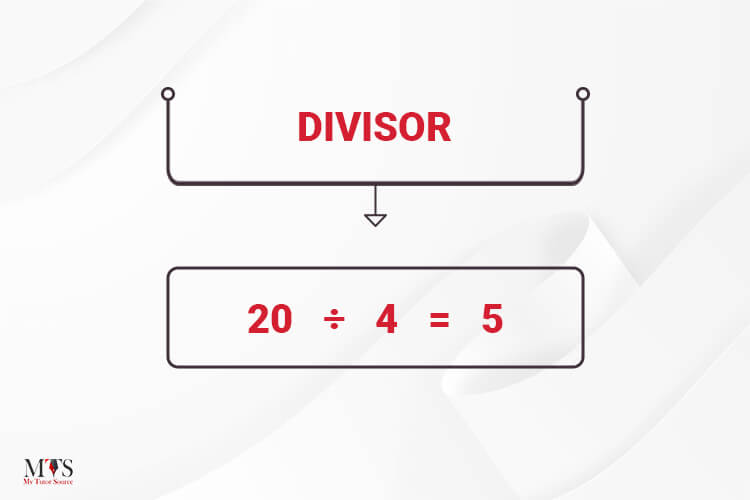In easy words, you can say that divisor is a number that attacks any number.

## What is Dividend?

Now, comes the dividend. The number that is being attacked by the divisor is called the dividend. Mathematically, the number that is being divided is called a dividend, as shown in the image below: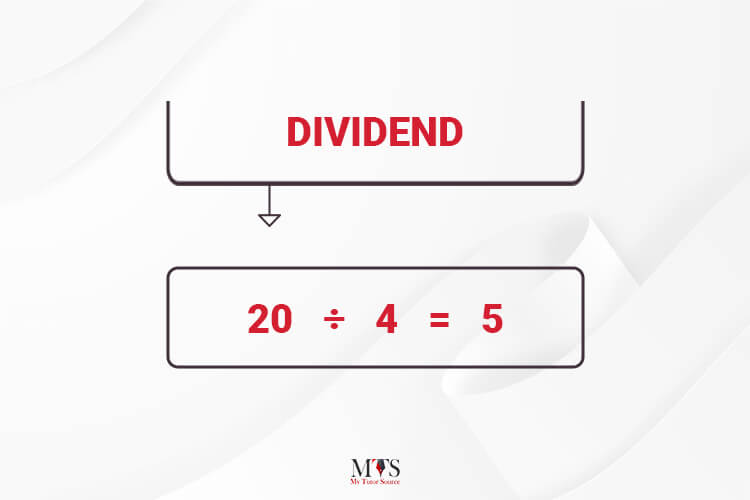Now, in this entire process, the number that we get as a result of dividing the dividend by the divisor is called the quotient.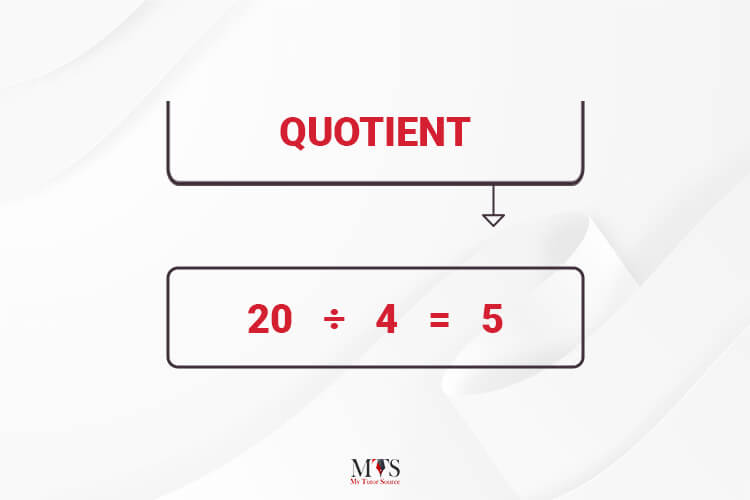Here is another way of writing the above equation: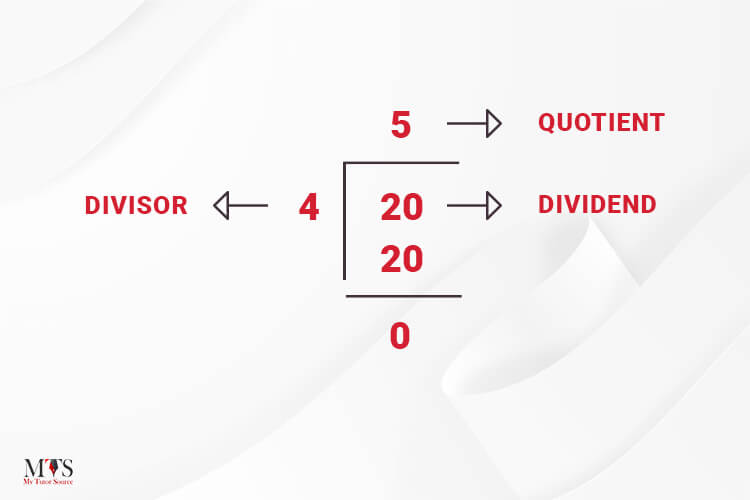## The Divisor and Dividend Formula!

In math, we’ve formulae for almost everything and divisor/dividend is no exception! You talk about the area of parallelograms or the interior angles of a polygon, math has standard formulae for all these terms.

The formula used for divisor and dividend is: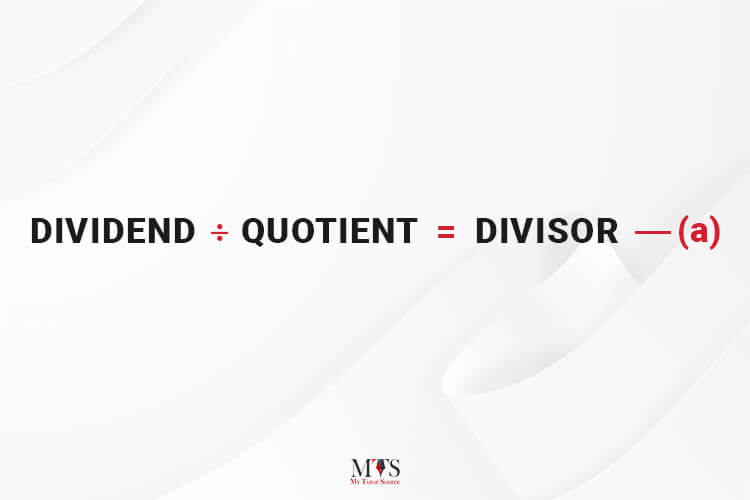The divisor can be brought to the L.H.S to find the dividend's value. But, according to the math rule, when something is being divided on the R.H.S and is taken to the L.H.S, it should be multiplied by the value on the L.H.S. So, you can also write the equation (a) as:

DIVIDEND = QUOTIENT × DIVISOR

## Divisors and the Factors of Numeric Numbers

As discussed above, the number that divides any digit is called a divisor. We can also call it a factor of that number. When finding the factors of an integral you can use the box-line method.

Let’s say, you want to find the factors of number 15, and write it on the top-left corner of the box-line (just like I did in the image below). Then, find the smallest number that could be divided by it. In this case, 3 is the smallest divisor that can be divided by 15. As a result, we get “5” as a quotient. Now, you’ve got to focus on the new quotient “5” to solve the equation further. 5 is the only smallest number that can be completely divided by the quotient “5”.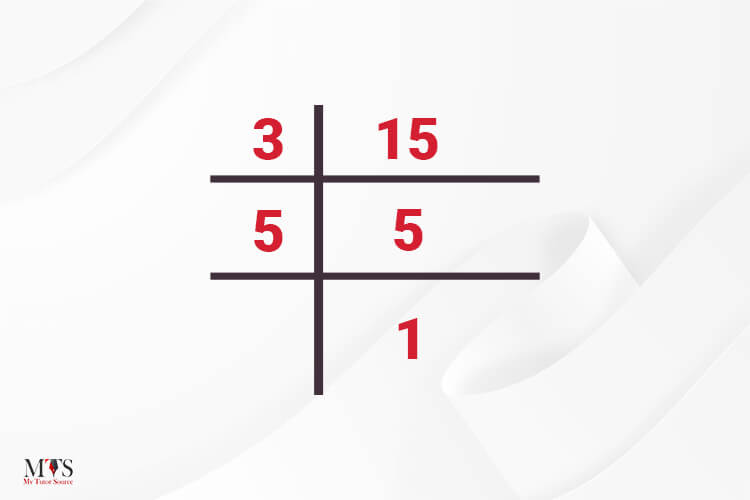Hence, the factors or divisors of 15 are 3 and 5.

The same method can be used for finding the factors of other integers.

Here you can check other examples of finding factors of a number: Factors of 24Factors of 36

## Key Points To Remember

• The divisor is responsible for dividing the number into parts.
• Divisor could be a negative or a positive number.
• When the divisor divides the number completely the remainder is always zero.
• When the divisor divides the number partially, the remainder is always a non-zero number.
• The divisors +1 and -1 can be divided by any number.

## FAQs

### Are divisors and dividends the same?

The divisors and factors are kind of the same. Divisors are digits that divide any number. On the other hand, factors are the divisors that divide the number and leave zero remainders.

### What’re the divisors of the number 18?

The divisors of 18 are 1, 2, 3, 6, 9, and 18.

### Can a divisor be negative?

Mathematically, negative divisors do exit. However, we usually consider the positive divisors.

### Find Top Tutors in Your AreaWith over 3 years of experience in teaching, Chloe is very deeply connected with the topics that talk about the educational and general aspects of a student's life. Her writing has been very helpful for students to gain a better understanding of their academics and personal well-being. I’m also open to any suggestions that you might have! Please reach out to me at chloedaniel402 [at] gmail.com